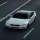Home
IT Knowledge
Inspiration
Languages
EN

# Java 8 - filter collections using stream

0 points
Created by:Brian-Tompset
431

In this article, we would like to show you how to filter collections using stream in Java 8.

## 1. Using lambda expression

In this example, we use stream `filter()` method with a lambda expression to make a list of bank accounts with more than 100 USD.

Practical example:

``````import java.io.*;
import java.util.ArrayList;
import java.util.List;
import java.util.stream.Collectors;

public class Example {

public static void main(String[] args) throws IOException {
BankAccount account1 = new BankAccount(1, 100);
BankAccount account2 = new BankAccount(2, 101);
BankAccount account3 = new BankAccount(3, 102);

List<BankAccount> accounts = new ArrayList<>();

List<BankAccount> accountsWithMoreThan100USD = accounts
.stream()
.filter(x -> x.getBalance() > 100)
.collect(Collectors.toList());

System.out.println(accountsWithMoreThan100USD);
}
}``````

Output:

``[BankAccount{ number=2, balance=101 USD }, BankAccount{ number=3, balance=102 USD }]``

## 2. Using method reference

In this example, we use stream `filter()` with method reference to make a list of bank accounts with more than 100 USD.

In this case, we need to add the following method to the `BankAccount` class:

``````public boolean hasMoreThan100USD() {
return this.balance > 100;
}``````

Practical example:

``````import java.io.*;
import java.util.ArrayList;
import java.util.List;
import java.util.stream.Collectors;

public class Example {

public static void main(String[] args) throws IOException {
BankAccount account1 = new BankAccount(1, 100);
BankAccount account2 = new BankAccount(2, 101);
BankAccount account3 = new BankAccount(3, 102);

List<BankAccount> accounts = new ArrayList<>();

List<BankAccount> accountsWithMoreThan100USD = accounts
.stream()
.filter(BankAccount::hasMoreThan100USD)
.collect(Collectors.toList());

System.out.println(accountsWithMoreThan100USD);
}
}``````

Output:

``[BankAccount{ number=2, balance=101 USD }, BankAccount{ number=3, balance=102 USD }]``

BankAccount.java:

``````public class BankAccount {
private final int accountNumber;
// for simplicity reason we keep USD in int
private final int balance;

public BankAccount(int accountNumber, int balance) {
this.accountNumber = accountNumber;
this.balance = balance;
}

public double getBalance() {
return balance;
}

public boolean hasMoreThan100USD() {
return this.balance > 100;
}

@Override
public String toString() {
return "BankAccount{ " +
"number=" + accountNumber +
", balance=" + balance +
" USD }";
}
}``````